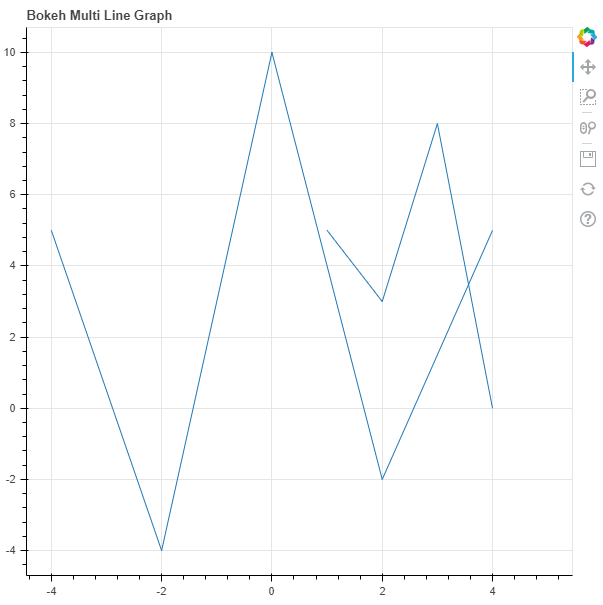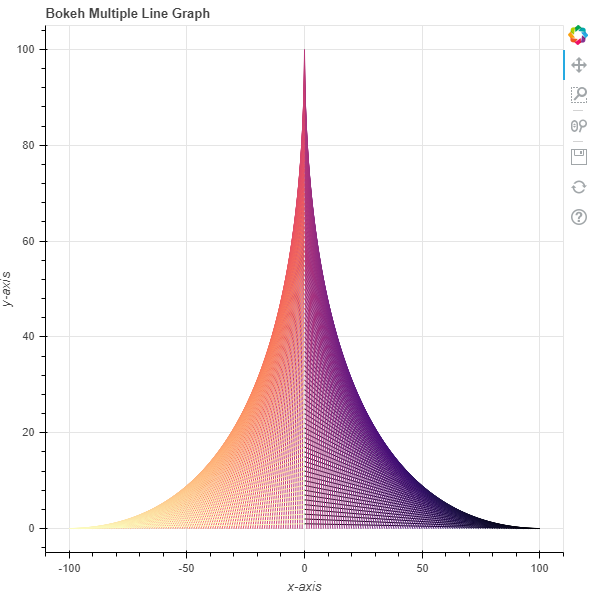Related Articles

# Python Bokeh – Plotting Multiple Lines on a Graph

• Last Updated : 10 Jul, 2020

Bokeh is a Python interactive data visualization. It renders its plots using HTML and JavaScript. It targets modern web browsers for presentation providing elegant, concise construction of novel graphics with high-performance interactivity.

Bokeh can be used to plot multiple lines on a graph. Plotting multiple lines on a graph can be done using the `multi_line()` method of the `plotting` module.

## plotting.figure.multi_line()

Syntax : multi_line(parameters)

Parameters :

• xs : x-coordinates of the lines
• ys : y-coordinates of the lines
• line_alpha : percentage value of line alpha, default is 1
• line_cap : value of line cap for the line, default is butt
• line_color : color of the line, default is black
• line_dash : value of line dash such as : solid, dashed, dotted, dotdash, dashdot [default is solid ]
• line_dash_offset : value of line dash offset, default is 0
• line_join : value of line join, default in bevel
• line_width : value of the width of the line, default is 1
• name : user-supplied name for the model
• tags : user-supplied values for the model

Other Parameters :

• alpha : sets all alpha keyword arguments at once
• color : sets all color keyword arguments at once
• legend_field : name of a column in the data source that should be used
• legend_group : name of a column in the data source that should be used
• legend_label : labels the legend entry
• muted : determines whether the glyph should be rendered as muted or not, default is False
• name : optional user-supplied name to attach to the renderer
• source : user-supplied data source
• view : view for filtering the data source
• visible : determines whether the glyph should be rendered or not, default is True
• x_range_name : name of an extra range to use for mapping x-coordinates
• y_range_name : name of an extra range to use for mapping y-coordinates
• level : specifies the render level order for this glyph

Returns : an object of class `GlyphRenderer`

Example 1 : In this example we will be using the default values for plotting the graph.

 `# importing the modules ``from` `bokeh.plotting ``import` `figure, output_file, show ``      ` `# file to save the model ``output_file(``"gfg.html"``) ``      ` `# instantiating the figure object ``graph ``=` `figure(title ``=` `"Bokeh Multi Line Graph"``) ``    ` `# the points to be plotted ``xs ``=` `[[``1``, ``2``, ``3``, ``4``, ``5``], [``-``4``, ``-``2``, ``0``, ``2``, ``4``]] ``ys ``=` `[[``5``, ``3``, ``8``, ``0``], [``5``, ``-``4``, ``10``, ``-``2``, ``5``]] ``   ` `# plotting the graph ``graph.multi_line(xs, ys) ``    ` `# displaying the model ``show(graph)`

Output :Example 2 : In this example we will be plotting the multiple lines with various other parameters

 `# importing the modules ``from` `bokeh.plotting ``import` `figure, output_file, show ``from` `bokeh.palettes ``import` `magma`` ` `# file to save the model ``output_file(``"gfg.html"``) ``      ` `# instantiating the figure object ``graph ``=` `figure(title ``=` `"Bokeh Multiple Line Graph"``) ``    ` `# name of the x-axis ``graph.xaxis.axis_label ``=` `"x-axis"``     ` `# name of the y-axis ``graph.yaxis.axis_label ``=` `"y-axis"``    ` `# the points to be plotted``x ``=` `[n ``for` `n ``in` `range``(``-``100``, ``101``)]``x.reverse()``xs ``=` `[[n, ``0``] ``for` `n ``in` `x]``y1 ``=` `[n ``for` `n ``in` `range``(``1``, ``101``)]``y1.reverse()``y ``=` `[n ``for` `n ``in` `range``(``1``, ``101``)] ``+` `[``0``] ``+` `y1``ys ``=` `[[``0``, n] ``for` `n ``in` `y] `` ` `# color of the lines``line_color ``=` `magma(``201``)``     ` `# plotting the graph ``graph.multi_line(xs, ys,``                 ``line_color ``=` `line_color) ``      ` `# displaying the model ``show(graph)`

Output :Attention geek! Strengthen your foundations with the Python Programming Foundation Course and learn the basics.

To begin with, your interview preparations Enhance your Data Structures concepts with the Python DS Course. And to begin with your Machine Learning Journey, join the Machine Learning – Basic Level Course

My Personal Notes arrow_drop_up